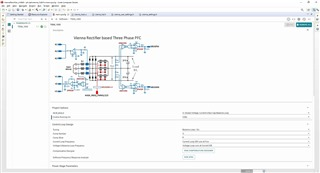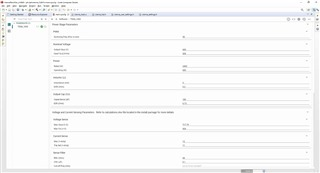If you have a related question, please click the "Ask a related question" button in the top right corner. The newly created question will be automatically linked to this question.

# TIDM-1000: PowerSUITE (main_syscfg) of Vienna rectifier-based three-phase PFC (TIDM-1000)

Part Number: TIDM-1000
Other Parts Discussed in Thread: POWERSUITE, C2000WARE, AMC1301

https://www.tij.co.jp/tool/jp/TIDM-1000

I purchased a Vienna rectifier and checked the operation.

Is there any material that explains PowerSUITE (main_syscfg) in detail?

For example, what is Operating (W) in the Power column of the attached image?

I would appreciate it if you could let me know if there are any sites or materials that explain in detail the upper and lower limits of Voltage Sence and Current Sence.• Hiroaki-san,

Please look at the calculations.xlsx sheet to understand the max and min value entry

C:\ti\c2000\C2000Ware_DigitalPower_SDK_3_02_00_00\solutions\tidm_1000\hardware

The values in the powerSUITE gui provide an easy way to modify the code for some limited parameters.

The powerSUITE tool uses the information to even model the system and uses this data in compensation designer to help design current compensator etc.

we have a small training material here

https://training.ti.com/solutions-fast-charging-electric-vehicle-supply-equipment-evse-design

Since I studied a little, I will write down what I understood for each item.

I would be grateful if you could point out or supplement what is wrong.

[Progect Options] INCR BUILD

This is an item to select the build type

[Progect Options] Control Running On

CPU method? Is there any problem with either?

[Control Loop Design] Tuning & Comp Number & Comp Style

It is automatically selected by the INCR BUILD number.

[Control Loop Design] Current Loop Frequency

I don't understand what this is.

[Control Loop Design] Voltage & Balance Loop Frequency

Since there is only one option, is this okay for the time being?

[PWM] Switching Freq (Fsw in kHz)

Is there a place to check the carrier frequency value, which has changed on the board?

[Nominal Voltage] Output Vbus (V)

Output voltage setting

[Nominal Voltage] Input Vl-L (Vrms)

Effective value of line voltage to be input

From tiducj0g.pdf

[Power] Rated (W)

The initial setting is 2400, but since "Input Vl-L (Vrms) = 208", do I need to change it to 1200? Confirmed that it works even under the conditions of "Input Vl-L (Vrms) = 208" and "Rated (W) = 2400".

[Power] Operating (W)

I don't understand what this is.

From 53PR116-292_110716.pdf

[Inductor (Li)] Inductance (mH) & DCR (Ohm)

Value of document "53PR116-292_110716.pdf", Inductance (mH) = 3 ≒ 2.92?

From tidrp45a.pdf

[Output Cap (C0)] Capacitance (uF) & ESR (Ohm)

The capacitor actually installed is 220uF, so you should change the initial value from 180 to 220, right? Along with that, ESR (Ohm) should be changed from 0.73 to 0.61 from the manufacturer's site, right?

calculations.xlsxより

[Voltage Sense] Max Vbus/2(V) = Vbus_max_sense

[Voltage Sense] Max Vin(+-V) = V range(+/-)

[Current sense] Max(+-Amp) = Imax_sense

[Current sense] Trip set(+-V) = ?

From tidrp45a.pdf

[Sence Filter] Rfiltr (Ohm) & Cfiltr (uF) & cut-off freq (kHz)

The value of the low-pass filter connected to the circuit of AMC1301

• ...

[Progect Options] INCR BUILD ,

This is an item to select the build type

[Progect Options] Control Running On

CPU method? Is there any problem with either?

<MB: You can keep it to C28x to begin with>

[Control Loop Design] Tuning & Comp Number & Comp Style

It is automatically selected by the INCR BUILD number.

[Control Loop Design] Current Loop Frequency

I don't understand what this is.

<MB This is the rate at which the current control loop will be run , typically specified at the rate of the PWM or half the rate of PWM etc>

[Control Loop Design] Voltage & Balance Loop Frequency

Since there is only one option, is this okay for the time being?

<MB Yes>

[PWM] Switching Freq (Fsw in kHz)

Is there a place to check the carrier frequency value, which has changed on the board?

<MB this is used to change the frequency setting, you can see the PWM by probing the signals on the board>

[Nominal Voltage] Output Vbus (V)

Output voltage setting

[Nominal Voltage] Input Vl-L (Vrms)

Effective value of line voltage to be input

From tiducj0g.pdf

[Power] Rated (W)

The initial setting is 2400, but since "Input Vl-L (Vrms) = 208", do I need to change it to 1200? Confirmed that it works even under the conditions of "Input Vl-L (Vrms) = 208" and "Rated (W) = 2400".

<MB I do not think this parameter is used , you can leave it at what it was>

[Power] Operating (W)

I don't understand what this is.

<MB This is the power level you will run the system at, the value is used only for the compensator design, the unit will work across the operating range>

From 53PR116-292_110716.pdf

[Inductor (Li)] Inductance (mH) & DCR (Ohm)

Value of document "53PR116-292_110716.pdf", Inductance (mH) = 3 ≒ 2.92?

From tidrp45a.pdf

[Output Cap (C0)] Capacitance (uF) & ESR (Ohm)

The capacitor actually installed is 220uF, so you should change the initial value from 180 to 220, right? Along with that, ESR (Ohm) should be changed from 0.73 to 0.61 from the manufacturer's site, right?

<MB you can change it , if will have a small impact on the compensator design>

calculations.xlsxより

[Voltage Sense] Max Vbus/2(V) = Vbus_max_sense

[Voltage Sense] Max Vin(+-V) = V range(+/-)

[Current sense] Max(+-Amp) = Imax_sense

[Current sense] Trip set(+-V) = ?

From tidrp45a.pdf

[Sence Filter] Rfiltr (Ohm) & Cfiltr (uF) & cut-off freq (kHz)

The value of the low-pass filter connected to the circuit of AMC1301